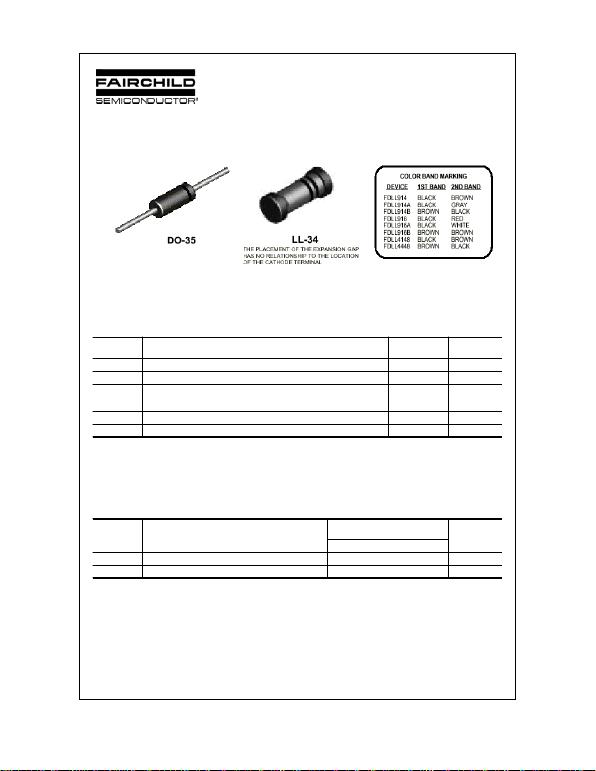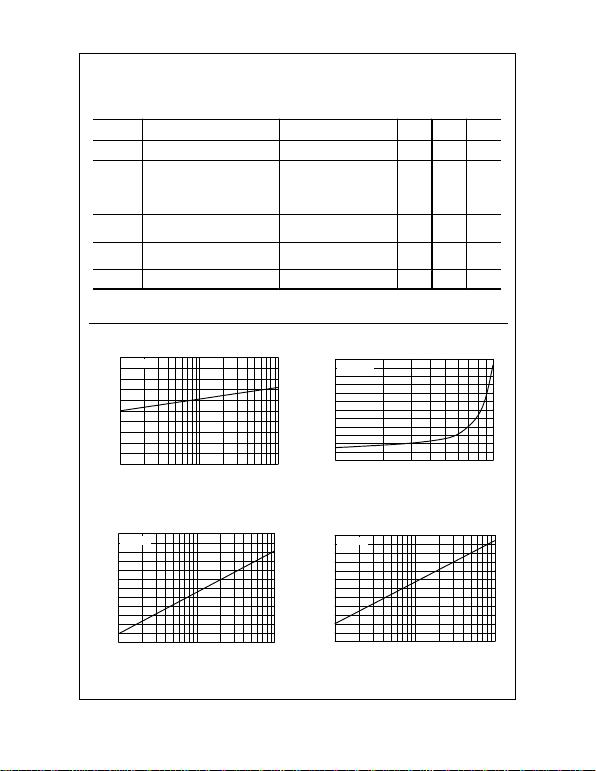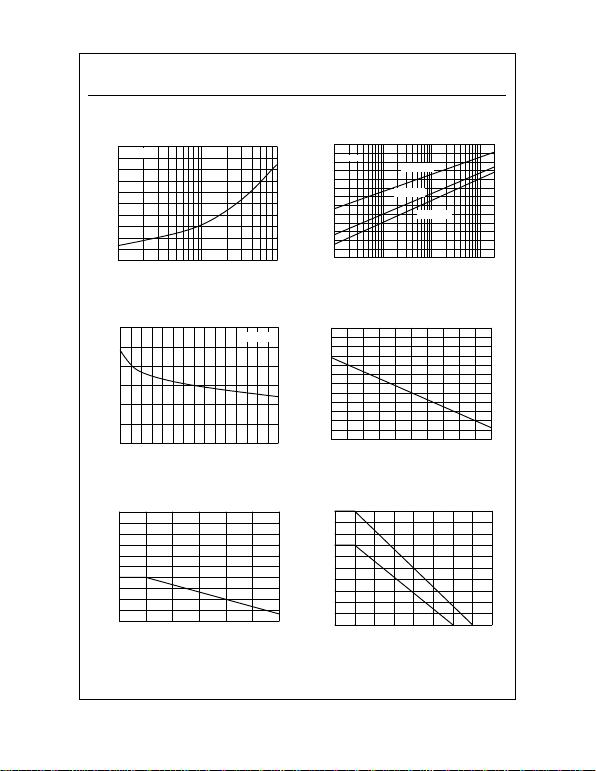1N/FDLL 914/A/B / 916/A/B / 4148 / 4448
1N/FDLL 914/A/B / 916/A/B / 4148 / 4448
Small Signal Diode
Absolute Maximum Ratings* TA = 25°C unless otherwise noted
*These ratings are limiting values above which the serviceability of any semiconductor device may be impaired.
NOTES:
1) These ratings are based on a maximum junction temperature of 200 degrees C.
2) These are steady state limits. The factory should be consulted on applications involving pulsed or low duty cycle operations.
Thermal Characteristics
2002 Fairchild Semiconductor Corporation
Symbol
Parameter
Value
Units
VRRM Maximum Repetitive Reverse Voltage 100 V
IF(AV) Average Rectified Forward Current 200 mA
IFSM Non-repetitive Peak Forward Surge Current
Pulse Width = 1.0 second
Pulse Width = 1.0 microsecond
1.0
4.0
A
A
Tstg Storage Temperature Range -65 to +200 °C
TJ Operating Junction Temperature 175 °C
Symbol
Characteristic
Max
Units
1N/FDLL 914/A/B / 4148 / 4448
PD Power Dissipation 500 mW
RθJA Thermal Resistance, Junction to Ambient 300 °C/W
1N/FDLL 914/A/B / 916/A/B / 4148 / 4448, Rev. B1N/FDLL 914/A/B / 916/A/B / 4148 / 4448
Typical Characteristics
Small Signal Diode
(continued)
Symbol
Parameter
Test Conditions
Min
Max
Units
VR Breakdown Voltage IR = 100 µA
IR = 5.0 µA 100
75 V
V
VF Forward Voltage 1N914B/4448
1N916B
1N914/916/4148
1N914A/916A
1N916B
1N914B/4448
IF = 5.0 mA
IF = 5.0 mA
IF = 10 mA
IF = 20 mA
IF = 20 mA
IF = 100 mA
620
630 720
730
1.0
1.0
1.0
1.0
mV
mV
V
V
V
V
IR Reverse Current VR = 20 V
VR = 20 V, TA = 150°C
VR = 75 V
25
50
5.0
nA
µA
µA
CT Total Capacitance
1N916A/B/4448
1N914A/B/4148
VR = 0, f = 1.0 MHz
VR = 0, f = 1.0 MHz
2.0
4.0
pF
pF
trr Reverse Recovery Time IF = 10 mA, VR = 6.0 V (60mA),
Irr = 1.0 mA, RL = 100 4.0 ns
Electrical Characteristics TA = 25°C unless otherwise noted
110
120
130
140
150
160 Ta=25 oC
1 2 3 5 10 20 30 5 0 100
Revers e Vo ltage, V
R [V]
Re verse Cu rrent, IR [uA]
Figure 1. Reverse V oltage vs Reverse Current
BV - 1.0 to 100 uA
0
20
40
60
80
100
120
10 2 0 3 0 50 70 100
Ta= 25 oC
Re verse Curre nt, I
R
[nA ]
Reverse Voltage, VR [V ]
Figure 2. Reverse Current vs Reverse V oltage
IR - 10 to 100 V
GENERAL RULE: The Reverse Current of a diode will approximately
double for every ten (10) Degree C increase in Temperature
250
300
350
400
450
500
550
1 2 3 5 10 20 3 0 50 100
Ta= 25 oC
Forward Voltage, V
R [mV]
Forward Current, IF [uA ]
Figure 3. Forward V oltage vs Forward Current
VF - 1 to 100 uA
450
500
550
600
650
700
750
0.1 0.2 0.3 0.5 1 2 3 5 1 0
Ta= 25 oC
Forw a rd V o lta g e, V
F [m V ]
Fo rwa rd C u rr en t, IF [mA ]
Figure 4. Forward V oltage vs Forward Current
VF - 0.1 to 10 mA1N/FDLL 914/A/B / 916/A/B / 4148 / 4448
Typical Characteristics (continued)
Small Signal Diode
(continued)
0 50 100 150
0
100
200
300
400
500
I
F(AV)
- AVERAGE RECTIFIED CURRENT - mA
Current (mA)
Ambient Tem perature ( oC)
02468101214
0.75
0.80
0.85
0.90
TA = 25 oC
Total Capacitance (pF)
REVERSE VOLTAGE (V)
0.6
0.8
1.0
1.2
1.4
1.6
10 20 30 50 100 200 300 500 800
Ta= 25 oC
Forward Voltage, V
F [mV]
Forward Current, IF [mA]
Figure 5. Forward V oltage vs Forward Current
VF - 10 to 800 mA
0.01 0.1 1 10
300
400
500
600
700
800
900
3
0.3
0.03
Typical
Ta= -40 oC
Ta= 25 oC
Ta= +65 oC
Forward Voltage, V
F [mV]
Forward Current, IF [mA]
Figure 6. Forward V oltage
vs Ambient Temperature
VF - 0.01 - 20 mA (-40 to +65 Deg C)
10 20 30 40 50 60
1.0
1.5
2.0
2.5
3.0
3.5
4.0
Ta = 25 oC
Reverse Recovery Time, t
rr [ns]
Reverse Recovery Current, Irr [mA ]
Figure 8. Reverse Recovery Time vs
Reverse Recovery Current
0 50 100 150 200
0
100
200
300
400
500
DO-35
SOT-23
Po wer Dissip ation, P
D [m W ]
Tem perature [ oC]
Figure 10. Power Derating Curve
Figure 7. T otal Capacitance IF = 10mA - IRR = 1.0 mA - Rloop = 100 Ohms
Figure 9. Average Rectified Current (IF(AV))
versus Ambient T emperature (TA)